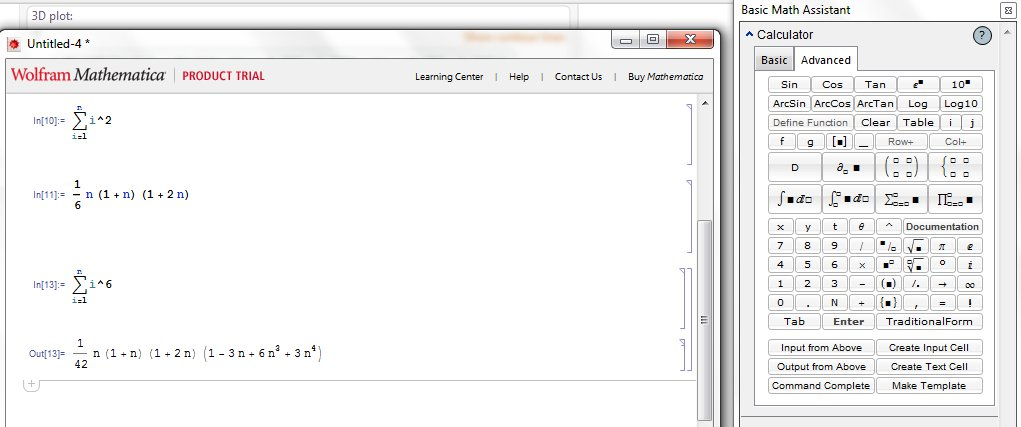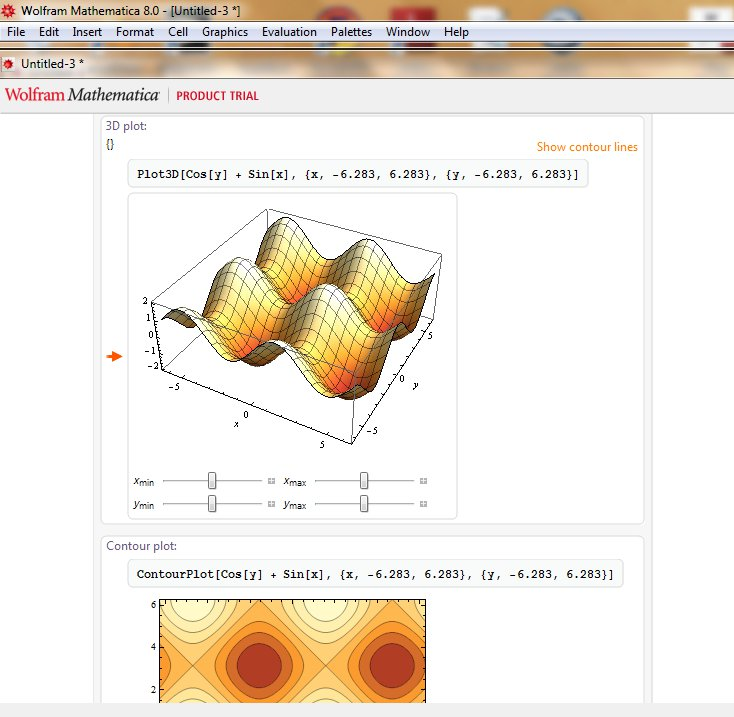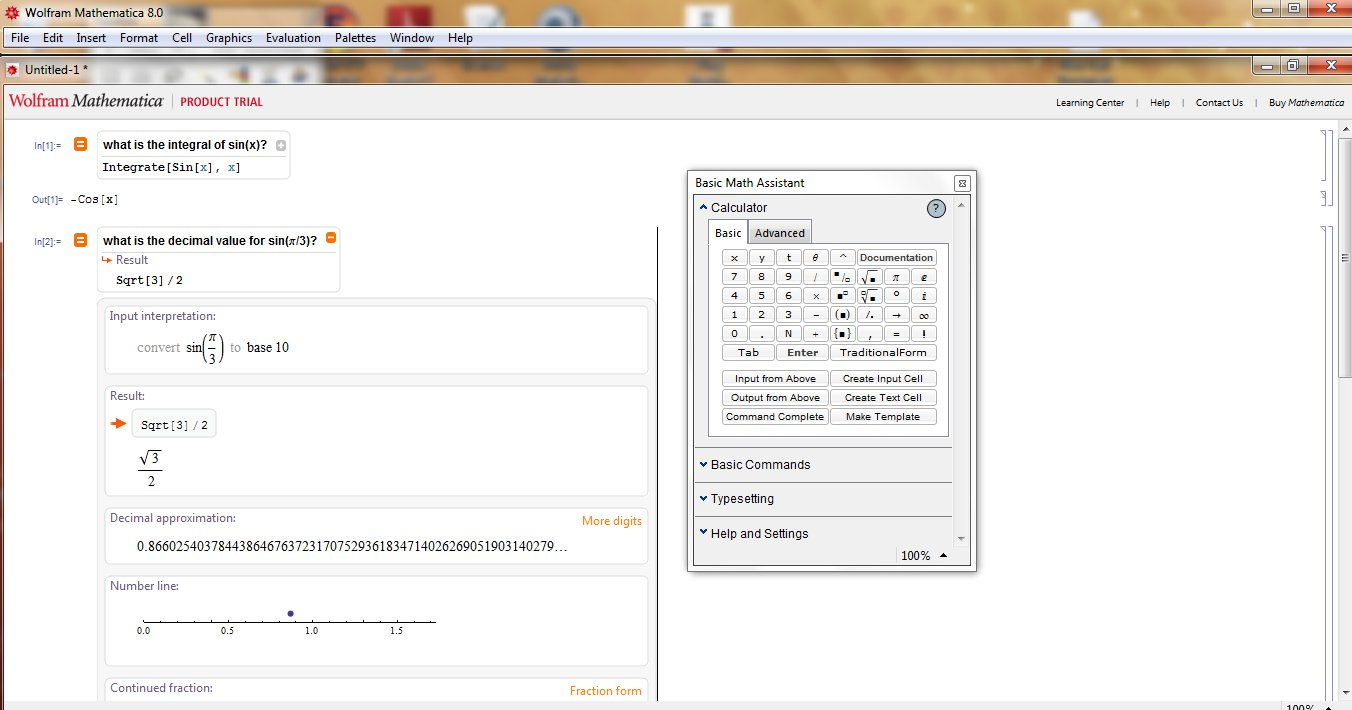# Mathematica Review

By Tech Powered Dad | February 2, 2011In 1986, Stephen Wolfram joined the faculty of the University of Illinois. He immediately began developing Mathematica, and it was released in 1988. By the time I arrived on the University of Illinois campus as a freshman in 1995, Wolfram was a local legend and Mathematica had become a part of the U of I math department culture. Curriculum and Mathematica (or C&M) sections of calculus were all the rage but somewhat controversial. Were students really learning calculus or were they merely learning to manipulate a computer?

Fifteen years later, the use of Mathematica 8 in the classroom causes less controversy. This incredible tool has become one of the most popular and powerful programs for the classroom. It puts hand held graphing calculators to shame as you can do far more than solve equations or plot with Mathematica.

There are already some reviews of Mathematica out there, but they are pretty technical. I wanted to do a Mathematica 8 review for the average high school or college student or teacher trying to decide if they want to buy a copy of Mathematica. SInce I am also a beginner on the software, I will keep my review as simple and non-technical as possible.

## What Is Mathematica?

First off, you need to understand that Mathematica’s abilities do go far beyond the classroom. Mathematica is a cutting edge software package that can calculate, graph, model, and present complex mathematical ideas that have applications from academic research to industry. The possible uses of Mathematica are endless, as you are limited only by your imagination to think of new mathematical questions to explore.For students, Mathematica is often used to explore subjects such as Calculus, Differential Equations, and Statistical Analysis, subjects often covered in depth in college, although advanced high school students could also tackle them. Mathematica gives students the ability to perform calculations that would be impractical or impossible using paper and pencil, opening up the possibility of a problems once previously inaccessible to high school and undergraduates (not to mention research mathematicians). Mathematica is capable of performing symbolic algebra calculations, much like a TI-Nspire CAS, but Mathematica’s computer algebra system is capable of far more sophisticated calculations that a handheld calculator would have no ability to solve. Mathematica can also model in a variety of ways from simple 2D plots to 3D color animations. It is set up to organize these calculations a “notebook,” slide show, or presentation.

One of the coolest thing about Mathematica 8 is called “free form input.” Mathematica inputs have gone through a revolution as its releases have progressed. In early versions, you had to learn the language of Mathematica in order to have the program say, solve an integral. As the software progressed, visual templates for integrals became available, allowing users to input an integral the same way they would appear in a textbook. With free form input, you can simply type your question in English, like “What is the integral of sin(x)?” This is amazing feature linguistic programming makes Mathematica accessible to beginners in a way that was unimaginable in that first release back in 1988.

Mathematica 8 also has access to all of the information in Wolfram Alpha. If you’ve never visited the Wolfram Alpha site, you should check it out. Not only do you have the ability to have Wolfram Alpha do some of the basic symbolic math that Mathematica has always done, but you can ask Wolfram Alpha an astonishing number of data related questions (try: “US population in 1787”). Now, Mathematica 8 has that ability integrated into it.

The answer to that question varies greatly depending on who you are. If you are a research mathematician or working in industry, this short review will probably not provide you with what you need to answer that question. My explanation of a few of the basic academic applications of Mathematica is kind of like saying, “Well, the best thing about computers is that they can do word processing,” when that’s truly only scratching the surface of what they can do. Needless to say, Mathematica has become an invaluable tool for mathematical research, and frankly, in ways that I as a humble high school math teacher can only begin to imagine.If you are a student or teacher, I can probably give you a a better answer. First, it’s hard to understate how helpful it is to have your own copy of Mathematica if you are a student taking a Mathematica integrated course or a teacher planning to teach or do demonstrations with it. Back when I was a college student, not every student even owned a computer, so students trekked off to “Mathematica labs” on campus. Now, you have the ability to have the power of Mathematica in your home, dorm room, or office.

Beyond official Mathematica courses, though, there’s no substitute for having this kind of tool for investigations, explorations, and checking your own ideas for understanding. Mathematica gives you the ability to quickly verify calculations, to explore possible patterns, create graphical representations, and far, far more. If you are a person who likes to think and play with ideas, you will quickly find yourself diving deeply in with Mathematica as you learn.

## Bottom Line Mathematica Review

This review only begins to describe what Mathematica can do, but again, my goal was simply to try to describe what Mathematica is to a beginner. And trying to describe Mathematica in a short review is next to impossible.  I’ve found it difficult to sum up the abilities of a TI-Nspire or Casio Prizm in a paragraph, and those pale in comparison with the abilities of a piece of software like Mathematica. While there have been competitors like Maple and Derive, Wolfram continues to be the leader with Mathematica. You can do more, explore more, and learn more with Mathematica. For the best price on a Mathematica download, you can buy Mathematica on Amazon.

STEM careers are the future. Will you be ready?